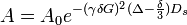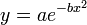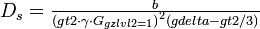# Water sLED pl.c (Pascale Legault)

This page is a part of the user-editable NMR Wiki pulse sequence database.

Disclaimer: You are solely responsible for correct use of these materials on your instrument.

Synopsis: measures diffusion of biomolecules in water

Family: Diffusion experiments

 Code authors: Pascale Legault

Copyright: P. Legault, lab webpage, University of Montreal

## Introduction

Amplitude of the echo follows the equation:$A = A_0 e^{-(\gamma \delta G)^2(\Delta-\frac{\delta}{3})D_s}$

Parameters translate in the following way (bold are vnmr parameters, units are in parentheses):

• Δ = gdelta (s)
• δ=gt2 (s)
• γ gyromagnetic ratio. For 1H γ = 2.675 222 099 x 104 (s-1 Gauss-1)
• G = gzlvl2 * gcal</sub> (Gauss)
• gcal is a calibration value that must be obtained for each probe

## Data processing

Fit the data to a simple equation:$y = a e^{-b x^2}$

It's a Gaussian curve with center at 0, where x = gzlvl2, y = peak intensity.

Then extract Ds from b:$\textstyle D_s = \frac{b}{{(gt2 \cdot \gamma \cdot G_{gzlvl2=1})}^2(gdelta-gt2/3)}$

Above, G(gzlvl2=1) is a probe-specific value of field gradient in Gauss per unit gzlvl2.

## References

1. Altieri, AS and Hinton, DP and Byrd, RA. Association of Biomolecular Systems via Pulsed Field Gradient NMR Self-Diffusion Measurements. Journal of the American Chemical Society 117(28):7566--7567, 1995. BibTeX [sled95]